Go to On Line Documents, Go to Go to Antique Computer home page

(Disclaimer) The text was scanned, OCRed, checked and presented here.
HOWEVER, the process is imperfect, and for serious work, the original should be used. (Links to the .gif images are at the top of each page.)

(added June 28, 2002) Thanks to LaFarr Stuart for a very careful proof reading and corrections. He is willing to try to explain the reasons for the procedures used here by Lehmer in simpler terms - "for the rest of us."

```
Lehmer, Chain Prime ..., starting page 00
Click here for GIF version of this page

```

# THE AMERICAN MATHEMATICAL MONTHLY

OFFICIAL JOURNAL OF

## THE MATHEMATICAL ASSOCIATION OF AMERICA

(INCORPORATED)

DEVOTED TO THE INTERESTS OF COLLEGIATE MATHEMATICS

EDITED BY

WILLIAM HENRY BUSSEY, Editor-in-Chief

HERBERT ELLSWORTH SLAUGHT
AUBREY JOHN KEMPNER

WITH THE COOPERATION OP
 H. E. BUCHANAN H. S. EVERETT C. N. MILLS ELIZABETH CARLSON B. F. FINKEL F. D. MURNAGHAN OTTO DUNKEL R. A. JOHNSON H. L. OLSON H. J. ETTLINGER H. W. KUHN D. E. SMITH

THE AMERICAN MATHEMATICAL MONTHLY, FOUNDED IN 109'! BY BENJAMIN F. FINKEL, WAS PUBLISHED BY HIM UNTIL 1913. From 1913 TO 1916 IT WAS OWNED AND PUBLISHED
BY REPRESENTATIVES OF FOURTEEN UNIVERSITIES AND COLLEGES
IN THE MIDDLE WEST

VOLUME XXXV

1928

PUBLISHED BY THE ASSOCIATION

MENASHA, WIS., AND MINNEAPOLIS, MINN.

```
Lehmer, Chain Prime ..., starting page 01
Click here for GIF version of this page

```

## THE MECHANICAL COMBINATION OF LINEAR FORMS

By D. H. LEHMER, University of California, Berkeley.

The "method of exclusion" introduced by Gauss, although a tentative method, is still one of the most powerful weapons for attacking many problems in the theory of numbers. Perhaps the most familar of such problems is the solution of the congruence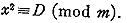The method is also of great use in the problem of representing a number by a given form such as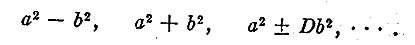Suppose, for instance, we wish to represent a given number N as the difference of two squares. We may choose 5 as an "excluding number." Let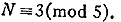We construct the following table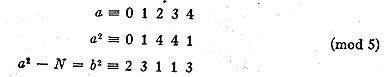But 2 and 3 are non-residues of 5 so that the cases d=0, 1, and 4 (mod 5) are excluded. We have then a =5n+2, 3. Other small prime moduli maybe used as excluding numbers with the result that a is restricted to (p ± 1)/2 values modulo p. The problem that now presents itself is the combination of the linear forms thus obtained. If the range of d is large, it is necessary to use many excluding numbers and the labor of combining directly these linear forms is prohibitive.

A graphical method has been suggested1 to solve this difficulty in which use is made of a table ruled in squares, each cell representing a possible value of a. "Movable strips" are made, the length of each being some excluding number p, and on which impossible
```
Lehmer, Chain Prime ..., starting page 02
Click here for GIF version of this page

```
values of a modulo p are indicated by crosses. These strips are moved down the columns of the table and the cells adjacent to the crosses on the strip are then ruled out. As many strips as are necessary are computed and applied until the number of empty cells is reasonably small for actual trial.

But even this method has certain disadvantages. In the first place it requires very close attention and much care. Also the strips are short in comparison with the length of the table and have to be shifted frequently, which is a possible source of error. Moreover the method makes it necessary to fix the limit of the table before commencing the work, so that the whole range must be entirely covered before any definite information can be obtained.

These difficulties are overcome by a machine constructed by the writer for the combination of linear forms. It is the object of this paper to give a brief description of the machine and to exhibit some of the results obtained by it.

Here instead of strips we have chains. The number of links in each chain is a convenient multiple of some small prime. In fact the chain lengths are: 64, 27, 25, 49, 22, 26, 17, 19, 23, 29, 31, 37, 41, 43, 47, 53, 59, 61, and 67. Each chain hangs in a loop from a sprocket having 10 teeth. All the 19 sprockets are fixed rigidly to a single shaft driven by a motor. The possible values modulo p are indicated by small pins fastened to the appropriate links of the chain (the zero link being indicated by red paint.) Whenever a link provided with a pin arrives at the top of the shaft a small spring with a tungsten point is lifted by the projecting pin. This breaks for the moment the electric contact between the spring and a brass bar running parallel to the shaft. By means of a relay in the circuit, the motor is shut off and the machine stops itself. When several chains are provided with springs the machine will not stop unless all the springs are lifted, so that every time the machine stops it means that a number satisfying all the imposed conditions has appeared. This number can be read directly by means of a revolution counter connected to the shaft. The shaft revolves 300 r. p. m. so that the machine canvasses 3000 numbers per minute. When all chains are provided with springs a "solution" occurs once in several hours during which time the machine runs without any attention.

The use of the machine is best explained by means of examples. Let us first consider the representation of
N = (1020 + 1)/(104 + 1) = 9999000099990001 = a2 - b2
as the difference of two squares. A representation besides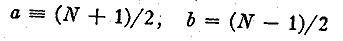```
Lehmer, Chain Prime ..., starting page 03
Click here for GIF version of this page

```
is possible since N has been shown to be the product of two primes.2 The factors of N are of the form 40n+1. The expansion of the square root of N in a regular continued fraction seems to indicate that, although 21 is a residue of N, 3 and 7 are both non-residues. The assumption that 3 is a non-residue gives the following form for the factors of N: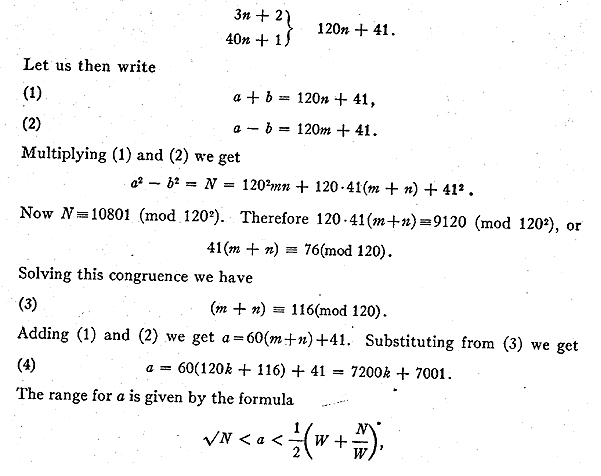where W is the limit to which the number has been searched for factors. In this case3 W =120,000 so that a has the range

99995000 < a < 41662560416 with 13888 < k < 5786466.

Now let x=k-13888 so that 0 < x < 5772578. Then (4) gives

```(5) 	a = 7200(x + 13888) + 7001 = 7200x + 100000601,
```
so that a is restricted to one case in 7200.
```
Lehmer, Chain Prime ..., starting page 04
Click here for GIF version of this page

```

We proceed now to exclude x with small prime moduli. Let us take for example the excluding number 11. We have from (5)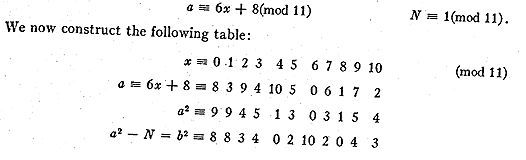Excluding those values of x which correspond to non-residues in the last line, we get the following linear forms for x modulo 11:

x = 11n + 2, 3, 4, 8, 9, 10.

Other excluding numbers may be dealt with in like manner.

Kraitchik4 has given tables of the possible values of a for any N and for excluding:- numbers =< 47. The possible values of x can easily be obtained from the tabulated values of a by means of the transformationThese tables have been recalculated and the errors noted are given at the end of the present paper. In order to serve the needs of the machine these tables have been extended to excluding numbers =< 67. The use of the tables is to be preferred to the method illustrated by the above example. The calculation is much simpler and the results can easily be checked by symmetry. For instance the table for 11 gives in our case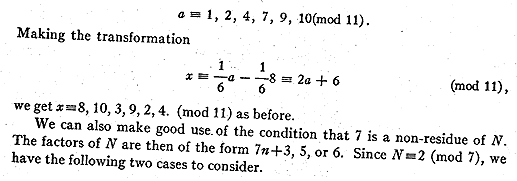```
Lehmer, Chain Prime ..., starting page 05
Click here for GIF version of this page

```Considering both cases we have

a = 49n + 2, 3,.9, 16, 23, 30, 37, 44.

Transforming to x we get

x = 49n + 2, 9, 16, 23, 25, 30, 37, 44.

We have thus restricted x to 8 cases modulo 49 'stead of the usual 28 cases. The result is that the machine will run longer between solutions.

Linear forms for x were thus calculated for every excluding number =< 67 and the appropriate links of each chain were supplied with pins. The machine was then set in motion. After running about 2 hours it stopped itself at x=400453,

a = 2983262201 ; a2 - N = 8889854359815374400 = 29815858802 = b2

This gives us N = (a - b) (a +b) =1676321 X 5964848081.

If the entire range had been covered without finding the factors of N, it would have indicated that 3 and 7 were residues contrary to our previous assumption. This case could have been taken care of, without changing the position of the pins, by simply shifting the origin on each chain as explained later. The machine would then have to be set back to zero and another run made. Even if. two runs were necessary the consideration of the quadratic character of 3 reduces the number of values of x to 2/9.

Another example of the representation by the difference of two squares is furnished by N=20191210335106439, a large factorof 3111+1. Every factor of the number is of the form 111n+1. Hence, as before, a can be restricted to one case in 1112=12321. Also a=4n+2. Consequently we have a=49284k+6550. The range for a is 44935627 < a < 10096101675 corresponding to W =100,000 as set by congruence tables. Hence
```
Lehmer, Chain Prime ..., starting page 06
Click here for GIF version of this page

```

```(5) 	a = 49284x + 44978200 with 0 < x < 203943 .
```

The linear forms for x were calculated as above with the exception of those for the excluding numbers 3 and 37 which are not prime to the modulus 49284. The first of these was computed as follows. The form (5) may be writtenwhere r designates all the quadratic residues of 9, which are 0, 1, 4, 7. Putting in these values we obtain

x = 9n + 1, 4, 7, 8.

The linear forms for 37 were calculated in a similar way. All the linear forms were set on the chains and the machine, after running only 4 seconds, stopped at x=145 giving

a = 52124380 b = 26414781.

Therefore N = 20191210335106439 = 25709599 X 78539161.

If the same problem had been attacked by the "movable strip" method, the entire range would have been excluded before this solution was discovered.

The machine was also used to show that the other large factor of 3111+1, namely 64326272436179833 is a prime which gives the complete factorisation

3111 + 1 = 22 X 7 X 223 X 18427 X 107671 X 25709599 X 56737873
X 78539161 X 64326272436179833.
The 5th and 7th of these primes are due to Poulet.

We consider next the representation of a number as the sum of two squares. The indeterminate equation x2-1721y2 = -1 has for its fundamental solution

x = 31738680901536260 y = 76506518214341.

We have then x2+1 =1721y2. Thus y is a divisor of the sum of two squares and therefore every factor of y is the sum of two squares. We have

y = 17 X 4500383424373 = 17 X N.

We propose to represent N as the sum of two squares. Using 5 as an excluding number we get a=5n+2, 3. To save time we consider the two separate cases a=5x+2 and a=5x+3. Taking the first case we proceed to exclude values of x with all the excluding numbers except 5. The tables constructed for the difference of squares may be used for the sum of squares. For an excluding number

```
Lehmer, Chain Prime ..., starting page 07
Click here for GIF version of this page

```
of the form 4n+1 the entries are identical. For the case 4n+3 the entries not tabulated are those desired, with the exception of the pair of entries corresponding toThe machine covered the range for the first case with but a single stop at

x = 196113, a = 980567, N - a2 = 3538871782884.

This number is not a square although it is a residue of every prime =<67.

To consider the second case, in which a=5x+3, no further calculation was necessary. The values of a in each case must be congruent modulo p. That isOn the second start therefore, instead of setting all the chains on their zero positions we set them on the link corresponding to the value of 1/5 (mod p). This time the machine stopped only once giving

x = 157044, a = 785223, b = 1970738. Hence N = (785223)2+(1970738)2.

Since this is a unique representation, it follows that N is a prime. This also gives us the complete factorisation of (31738680901536260)2+1.

By separating the work into two cases, results were obtained in 2/5 of the time required for a single run.

The machine has been used to study the following problem previously considered by Kraitchik,5 namely to find the least non-square which is a quadratic residue of all primes =< p.

Following Kraitchik, we consider 0 as a non-residue-and the number 8 instead of the prime 2. The numbers sought belong to the forms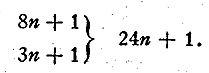Letting N=24x+l we proceed to exclude values of x with moduli =>5. To obtain the necessary linear forms we transform a table of residues by means of the relationwhere y represents the residues of p: All the chains were supplied with pins before starting. To start with, the chain for 5 was provided with a spring and the machine was set in motion. The first non-square solution obtained was 241. Then the next prime was introduced by setting on the spring of the chain for 7. The machine was then run to the first non-square solution after which the spring for the prime 11 was put on,
```
Lehmer, Chain Prime ..., starting page 08
Click here for GIF version of this page

```
etc. The squares appearing as solutions are of course squares of primes or products of primes >p. These squares were predicted in advance so as to avoid unnecessary reading of the machine. The work was carried up to p=61. The results are summarized in the following table, which gives the least nonsquare NP that is a residue of all primes < p.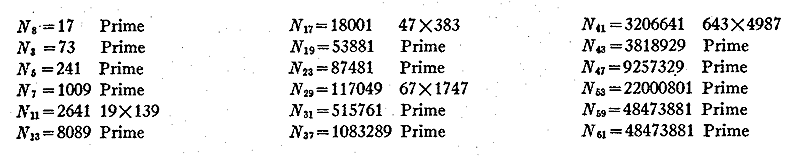It is seen that most of the N's are primes. If NP is a prime it has for residues all the primes =< p and also -1. If it is composite there exists some prime =< p which is a non-residue of an even number of factors of N. The interesting case of N59=N61, is the first of its kind.

The above results confirm and extend the work of Kraitchik. He used the movable strip method with a table having 180 columns and 84 lines to carry the work to N47. A full account of his work is given in his book. A few errors are to be noted which however do not happen to influence the final results. It should be pointed out that to extend Kraitchik's work by his method would imply the construction of an entirely new table the size of which must be determined in advance. Using the machine the work may be extended indefinitely and is complete as far as it goes.

## ERRORS IN KRAITCHIK'S TABLE III.

Theorie des Nombres, pp. 190-9
```p	N	for	read
31	5	a=8	a=11
31	5	t = 11	t = 8
47	6	t = 10	t = 11
47	34	t = 19	t = 22
```
Since writing the above, the author's attention has been directed to Kraitchick's new second volume (Theorie des Nombres, vol. 2, Paris, 1926) which contains tables of a (mod p) for p =< 67. The above errors have been corrected. Only two new error, appear, namely:
```p	N	for	read
59	23	x=14 	x=15
59	44	x=14	x=17
```

1. Kraitchik, Theorie des Nombres (Paris, 1922), Chapter 2.
2. Bulletin of the American Mathematical Society, vol. 33, p. 336.
3. This limit has been established by Shanks' table of the number of digits in the repetend of the reciprocal of every prime<120000.
4. Kraitchik, loc. cit., pp. 187-199.
5. Recherches sur la Theorie des Nombres (Paris, 1924), pp. 41-46.

Go to On Line Documents, Go to Go to Antique Computer home page